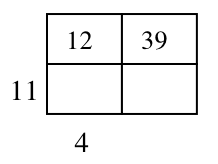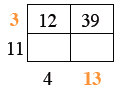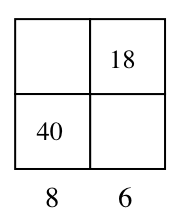### Home > INT2 > Chapter 1 > Lesson 1.3.2 > Problem1-88

1-88.

Determine the total area of each rectangle below. Each number inside the rectangle represents the area of that smaller rectangle, while each number along the side represents the length of that portion of the side.

1.Find the sides that you know from the products within the boxes.
$12\div4=3$
$39\div3=13$

Now find the areas of all of the boxes inside the rectangle.
Add them together for the total area of the rectangle.
$12+39+44+143$

$238$

1.Use the same strategy for part (b).